# Python Break 和 Continue 语句

## 如何在 Python 中使用 break 语句

``````for letter in 'freeCodeCamp':
print('letter :', letter)
``````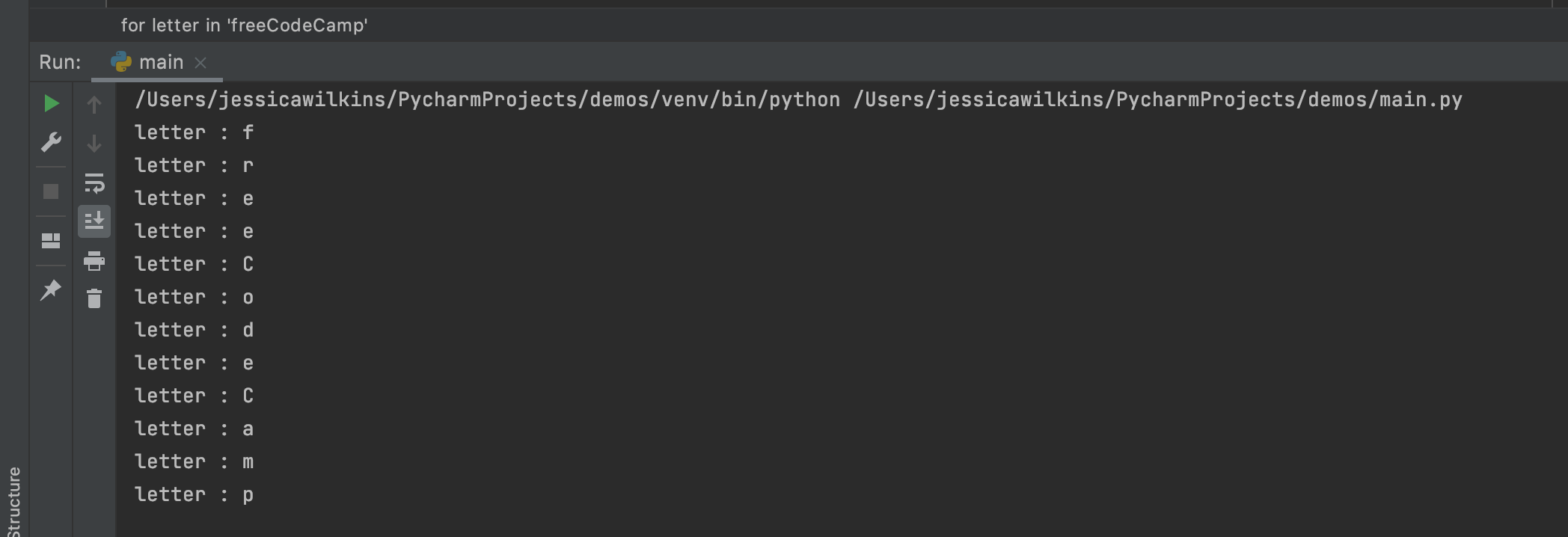``````for letter in 'freeCodeCamp':
if letter == "o":
break
print('letter :', letter)
``````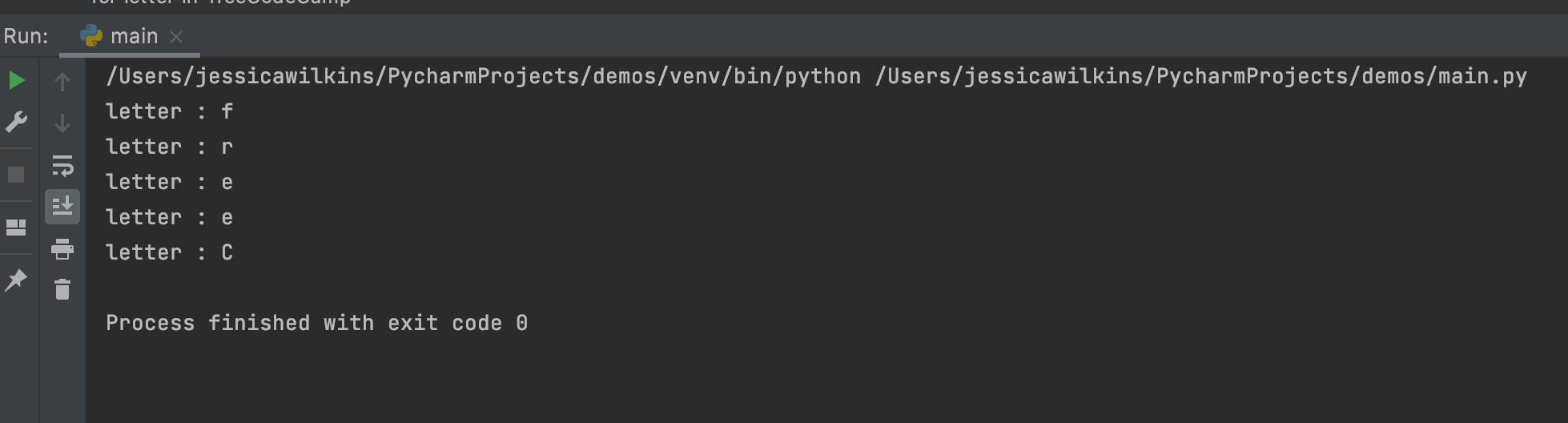``````num = 5
while num < 20:
print('Current number :', num)
num = num + 1
``````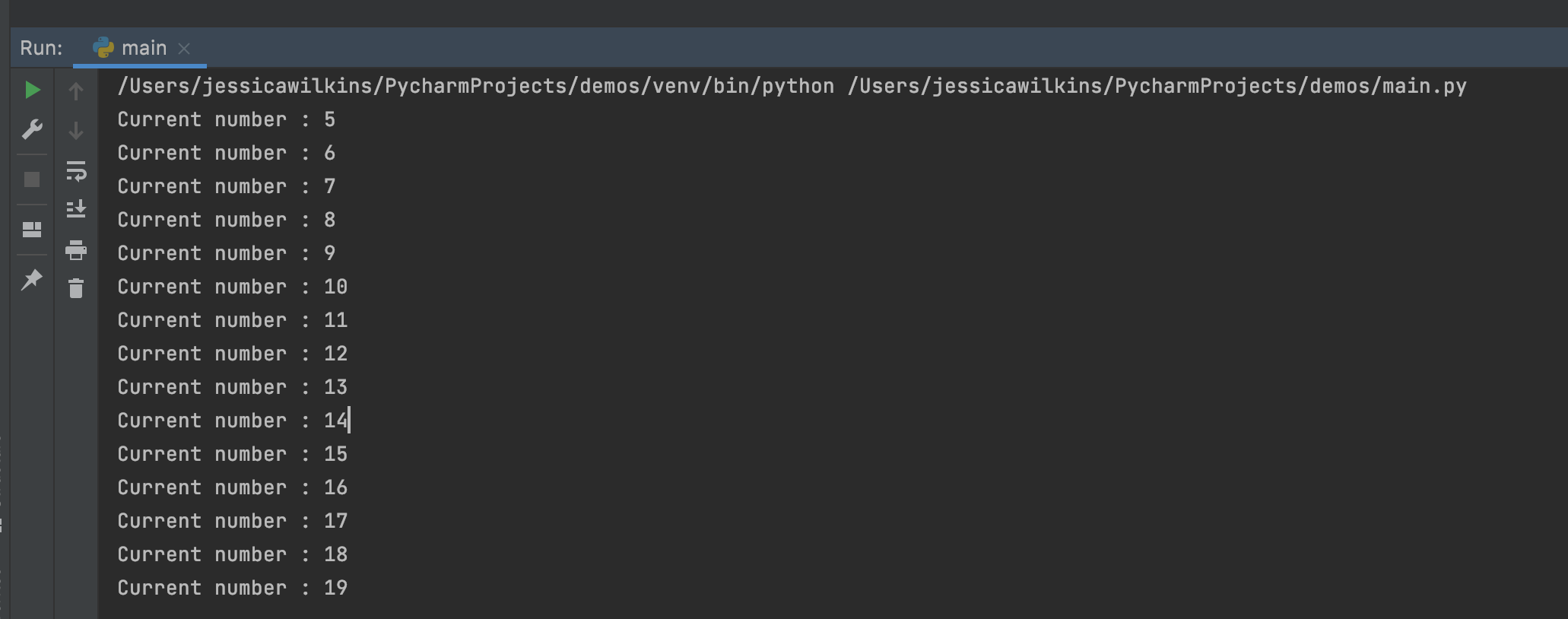``````num = 5
while num < 20:
print('Current number :', num)
num = num + 1
if num == 9:
break
``````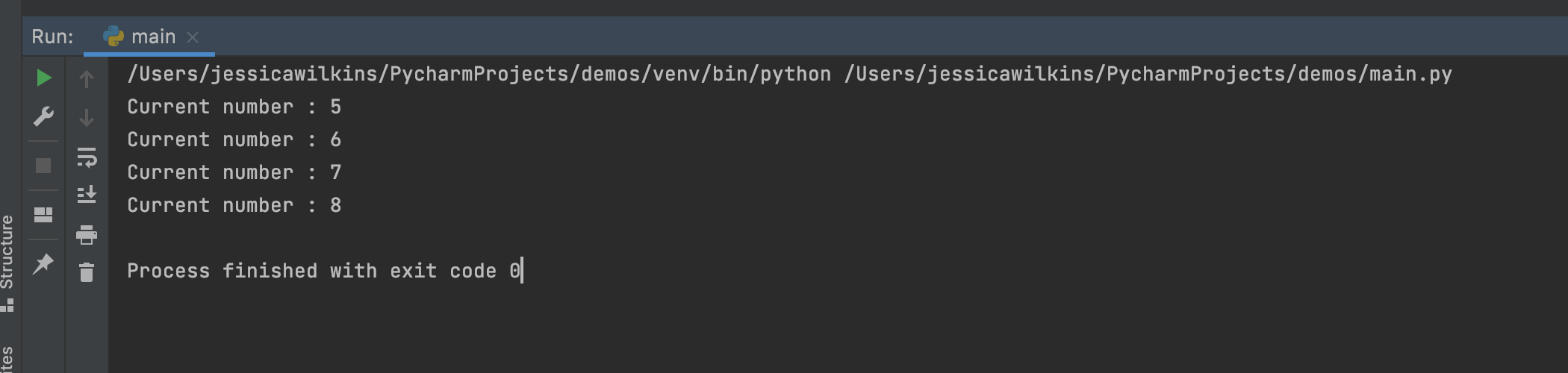## 如何在 Python 中使用 continue 语句

``````for letter in "Jessica":
``````

``````  if letter == "i":
continue
``````

``````for letter in "Jessica":
if letter == "i":
continue
print(letter)
``````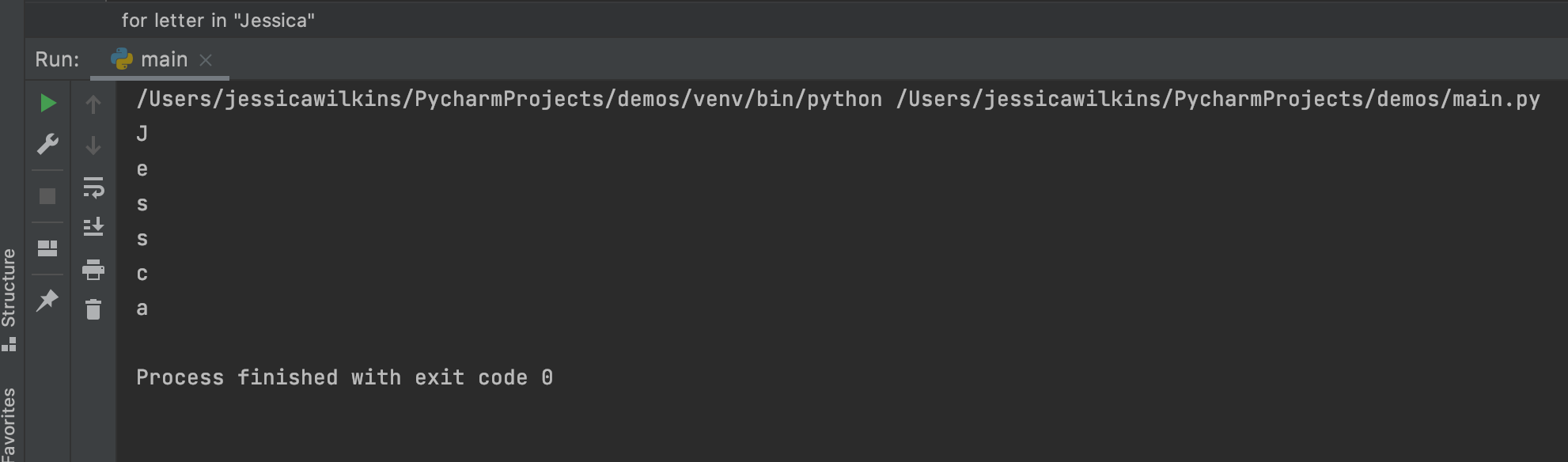``````num = 10
while num < 100:
num = num + 10
if num == 50:
continue
print("Current num: ", num)
``````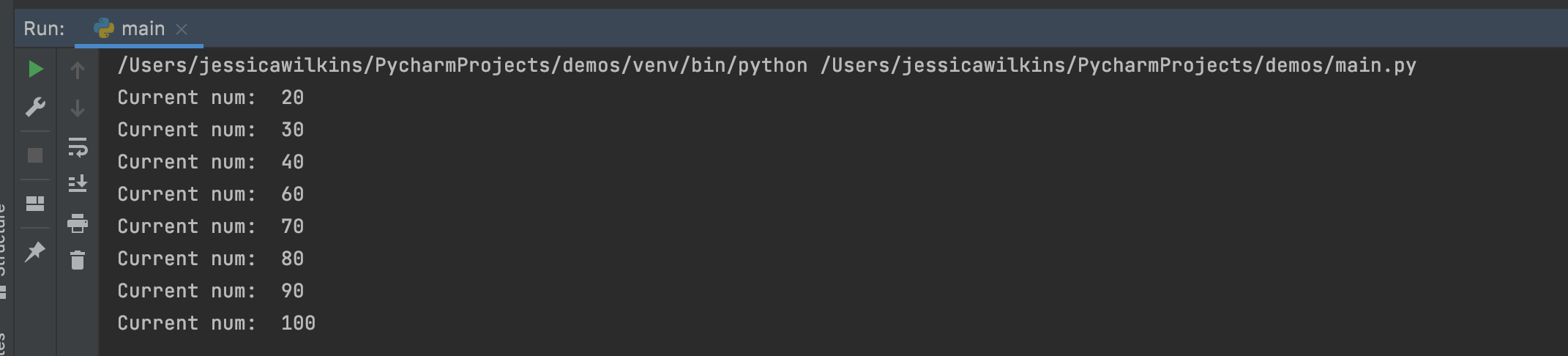## 总结

Python 中的 `break` 和 `continue` 语句是用来跳过当前循环的一部分或完全脱离循环的。